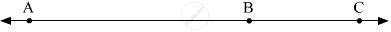Advertisement Remove all ads

# Answer the Following Question. If a - B - C And D(A,C) = 17, D(B,C) = 6.5 Then D(A,B) = ? - Geometry

Sum

Answer the following question.

If A - B - C and d(A,C) = 17, d(B,C) = 6.5 then d(A,B) = ?

Advertisement Remove all ads

#### SolutionWe have, d(A, C) = 17; d(B, C) = 6.5

Now, d(A, C) = d(A, B) + d(B, C)

So, d(A, B) = d(A, C) − d(B, C) = 17 − 6.5

∴ d(A, B) = 10.5

Concept: Co-ordinates of Points and Distance
Is there an error in this question or solution?
Advertisement Remove all ads

#### APPEARS IN

Balbharati Mathematics 2 Geometry 9th Standard Maharashtra State Board
Chapter 1 Basic Concepts in Geometry
Problem set 1 | Q 5.1 | Page 12
Advertisement Remove all ads
Advertisement Remove all ads
Share
Notifications

View all notifications

Forgot password?